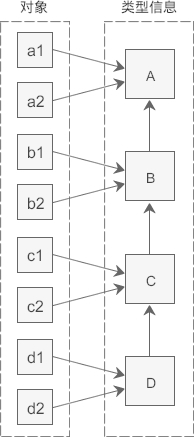## C++ static_cast、dynamic_cast、const_cast和reinterpret_cast（四种类型转换运算符）

• 内容
• 评论
• 相关

static_cast 用于良性转换，一般不会导致意外发生，风险很低。
const_cast 用于 const 与非 const、volatile 与非 volatile 之间的转换。
reinterpret_cast 高度危险的转换，这种转换仅仅是对二进制位的重新解释，不会借助已有的转换规则对数据进行调整，但是可以实现最灵活的 C++ 类型转换。
dynamic_cast 借助 RTTI，用于类型安全的向下转型（Downcasting）。

xxx_cast<newType>(data)

newType 是要转换成的新类型，data 是被转换的数据。例如，老式的C风格的 double 转 int 的写法为：

```double scores = 95.5;
int n = (int)scores;```

C++ 新风格的写法为：

```double scores = 95.5;
int n = static_cast<int>(scores);```

## static_cast 关键字

static_cast 只能用于良性转换，这样的转换风险较低，一般不会发生什么意外，例如：

• 原有的自动类型转换，例如 short 转 int、int 转 double、const 转非 const、向上转型等；
• void 指针和具体类型指针之间的转换，例如`void *``int *``char *``void *`等；
• 有转换构造函数或者类型转换函数的类与其它类型之间的转换，例如 double 转 Complex（调用转换构造函数）、Complex 转 double（调用类型转换函数）。

• 两个具体类型指针之间的转换，例如`int *``double *``Student *``int *`等。不同类型的数据存储格式不一样，长度也不一样，用 A 类型的指针指向 B 类型的数据后，会按照 A 类型的方式来处理数据：如果是读取操作，可能会得到一堆没有意义的值；如果是写入操作，可能会使 B 类型的数据遭到破坏，当再次以 B 类型的方式读取数据时会得到一堆没有意义的值。
• int 和指针之间的转换。将一个具体的地址赋值给指针变量是非常危险的，因为该地址上的内存可能没有分配，也可能没有读写权限，恰好是可用内存反而是小概率事件。

static_cast 也不能用来去掉表达式的 const 修饰和 volatile 修饰。换句话说，不能将 const/volatile 类型转换为非 const/volatile 类型。

static_cast 是“静态转换”的意思，也就是在编译期间转换，转换失败的话会抛出一个编译错误。

```#include <iostream>
#include <cstdlib>
using namespace std;

class Complex{
public:
Complex(double real = 0.0, double imag = 0.0): m_real(real), m_imag(imag){ }
public:
operator double() const { return m_real; }  //类型转换函数
private:
double m_real;
double m_imag;
};

int main(){
//下面是正确的用法
int m = 100;
Complex c(12.5, 23.8);
long n = static_cast<long>(m);  //宽转换，没有信息丢失
char ch = static_cast<char>(m);  //窄转换，可能会丢失信息
int *p1 = static_cast<int*>( malloc(10 * sizeof(int)) );  //将void指针转换为具体类型指针
void *p2 = static_cast<void*>(p1);  //将具体类型指针，转换为void指针
double real= static_cast<double>(c);  //调用类型转换函数

//下面的用法是错误的
float *p3 = static_cast<float*>(p1);  //不能在两个具体类型的指针之间进行转换
p3 = static_cast<float*>(0X2DF9);  //不能将整数转换为指针类型

return 0;
}```

## const_cast 关键字

const_cast 比较好理解，它用来去掉表达式的 const 修饰或 volatile 修饰。换句话说，const_cast 就是用来将 const/volatile 类型转换为非 const/volatile 类型。

```#include <iostream>
using namespace std;

int main(){
const int n = 100;
int *p = const_cast<int*>(&n);
*p = 234;
cout<<"n = "<<n<<endl;
cout<<"*p = "<<*p<<endl;

return 0;
}```

n = 100
*p = 234

`&n`用来获取 n 的地址，它的类型为`const int *`，必须使用 const_cast 转换为`int *`类型后才能赋值给 p。由于 p 指向了 n，并且 n 占用的是栈内存，有写入权限，所以可以通过 p 修改 n 的值。

cout<<"n = "<<100<<endl;

## reinterpret_cast 关键字

reinterpret 是“重新解释”的意思，顾名思义，reinterpret_cast 这种转换仅仅是对二进制位的重新解释，不会借助已有的转换规则对数据进行调整，非常简单粗暴，所以风险很高。

reinterpret_cast 可以认为是 static_cast 的一种补充，一些 static_cast 不能完成的转换，就可以用 reinterpret_cast 来完成，例如两个具体类型指针之间的转换、int 和指针之间的转换（有些编译器只允许 int 转指针，不允许反过来）。

```#include <iostream>
using namespace std;

class A{
public:
A(int a = 0, int b = 0): m_a(a), m_b(b){}
private:
int m_a;
int m_b;
};

int main(){
//将 char* 转换为 float*
char str[]="http://c.biancheng.net";
float *p1 = reinterpret_cast<float*>(str);
cout<<*p1<<endl;
//将 int 转换为 int*
int *p = reinterpret_cast<int*>(100);
//将 A* 转换为 int*
p = reinterpret_cast<int*>(new A(25, 96));
cout<<*p<<endl;

return 0;
}```

3.0262e+29
25

## dynamic_cast 关键字

dynamic_cast 用于在类的继承层次之间进行类型转换，它既允许向上转型（Upcasting），也允许向下转型（Downcasting）。向上转型是无条件的，不会进行任何检测，所以都能成功；向下转型的前提必须是安全的，要借助 RTTI 进行检测，所有只有一部分能成功。

dynamic_cast 与 static_cast 是相对的，dynamic_cast 是“动态转换”的意思，static_cast 是“静态转换”的意思。dynamic_cast 会在程序运行期间借助 RTTI 进行类型转换，这就要求基类必须包含虚函数；static_cast 在编译期间完成类型转换，能够更加及时地发现错误。

dynamic_cast 的语法格式为：

dynamic_cast <newType> (expression)

newType 和 expression 必须同时是指针类型或者引用类型。换句话说，dynamic_cast 只能转换指针类型和引用类型，其它类型（int、double、数组、类、结构体等）都不行。

#### 1) 向上转型（Upcasting）

「向上转型时不执行运行期检测」虽然提高了效率，但也留下了安全隐患，请看下面的代码：

```#include <iostream>
#include <iomanip>
using namespace std;

class Base{
public:
Base(int a = 0): m_a(a){ }
int get_a() const{ return m_a; }
virtual void func() const { }
protected:
int m_a;
};

class Derived: public Base{
public:
Derived(int a = 0, int b = 0): Base(a), m_b(b){ }
int get_b() const { return m_b; }
private:
int m_b;
};

int main(){
//情况①
Derived *pd1 = new Derived(35, 78);
Base *pb1 = dynamic_cast<Derived*>(pd1);
cout<<"pd1 = "<<pd1<<", pb1 = "<<pb1<<endl;
cout<<pb1->get_a()<<endl;
pb1->func();

//情况②
int n = 100;
Derived *pd2 = reinterpret_cast<Derived*>(&n);
Base *pb2 = dynamic_cast<Base*>(pd2);
cout<<"pd2 = "<<pd2<<", pb2 = "<<pb2<<endl;
cout<<pb2->get_a()<<endl;  //输出一个垃圾值
pb2->func();  //内存错误

return 0;
}```

`pb2->func()`得不到 func() 的正确地址的原因在于，pb2 指向的是一个假的“对象”，它没有虚函数表，也没有虚函数表指针，而 func() 是虚函数，必须到虚函数表中才能找到它的地址。

#### 2) 向下转型（Downcasting）

```#include <iostream>
using namespace std;

class A{
public:
virtual void func() const { cout<<"Class A"<<endl; }
private:
int m_a;
};

class B: public A{
public:
virtual void func() const { cout<<"Class B"<<endl; }
private:
int m_b;
};

class C: public B{
public:
virtual void func() const { cout<<"Class C"<<endl; }
private:
int m_c;
};

class D: public C{
public:
virtual void func() const { cout<<"Class D"<<endl; }
private:
int m_d;
};

int main(){
A *pa = new A();
B *pb;
C *pc;

//情况①
pb = dynamic_cast<B*>(pa);  //向下转型失败
if(pb == NULL){
cout<<"Downcasting failed: A* to B*"<<endl;
}else{
cout<<"Downcasting successfully: A* to B*"<<endl;
pb -> func();
}
pc = dynamic_cast<C*>(pa);  //向下转型失败
if(pc == NULL){
cout<<"Downcasting failed: A* to C*"<<endl;
}else{
cout<<"Downcasting successfully: A* to C*"<<endl;
pc -> func();
}

cout<<"-------------------------"<<endl;

//情况②
pa = new D();  //向上转型都是允许的
pb = dynamic_cast<B*>(pa);  //向下转型成功
if(pb == NULL){
cout<<"Downcasting failed: A* to B*"<<endl;
}else{
cout<<"Downcasting successfully: A* to B*"<<endl;
pb -> func();
}
pc = dynamic_cast<C*>(pa);  //向下转型成功
if(pc == NULL){
cout<<"Downcasting failed: A* to C*"<<endl;
}else{
cout<<"Downcasting successfully: A* to C*"<<endl;
pc -> func();
}

return 0;
}```

Downcasting failed: A* to B*
Downcasting failed: A* to C*
-------------------------
Downcasting successfully: A* to B*
Class D
Downcasting successfully: A* to C*
Class D0条评论